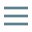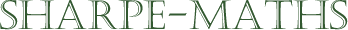# Who was responsible for the letters in mathematics?

The who is way less important here than the why?

The Arabian mathematician & all round genius, Muhammad ibn Musa al-Khwarizmi was the guy who really got the ball rolling. The title of his book contains the word “Al jabr” which is where algebra comes from etimologically. His name has been corrupted somewhat to give us the word algorithm, so he's a very important dude.

His work was picked up by a chap called Leonardo of Pisa (no relation) who is more commonly known by his nickname, Fibonnacci. He brought the ideas to Europe which helped started the Renaissance in Europe.

With that out of the way, let's now ask why?

Consider the set of all additions of whole numbers. 1 + 2 = 3, 1 + 3 = 4 etc.. It is clear after you think about this for more than a millisecond, that the list is going to be long. Very long. In fact, it is infinitely long. You will never complete it. So don't try.

Now let's take a simple statement. If you add two odd numbers together, you will always get an even number.

The problem for the mathematician is how to prove this. To prove a statement, you must show that it works in ALL CASES.

But we have already found that this is a never ending process, as however many examples we try:

1 + 1 = 2 ✅

1 + 3 = 4 ✅

1 + 5 = 6 ✅ …

However long we continue in this way, we will never reach the end.

But what if we could write down an expression which represents ALL CASES. $x + y$ works to represent all possible numbers & their sums, but we have a problem.

We have no idea if $x$ or $y$ or both or neither are odd numbers. Here's the good bit. Let us suppose that x represents all whole numbers (these are known as the Natural numbers) then $2x$ is always going to represent an even number and if we know an even number is $2x$ then the next odd number will be $2x + 1$ & the previous one will be $2x - 1$. So let's now make up our expressions for ANY 2 odd numbers as

$2x - 1$ and $2y - 1$ (I could use $2x + 1$ but with counting numbers, this will exclude the number 1)

Now we have a representation of our two odd numbers, let's add them together

\begin{aligned}&2x - 1 + 2y - 1 \\&{= 2x + 2y - 2}\\&{= 2(x + y - 1)} \end{aligned}

This is clearly even because it is a multiple of 2. Therefore our statement is true. Which is a relief.

This is why algebra is awesome & some of us maths geeks get very excited by its possibilities.

Problems that were impossible to solve, are now possible & if that isn't creative thought, I'm not sure what is.